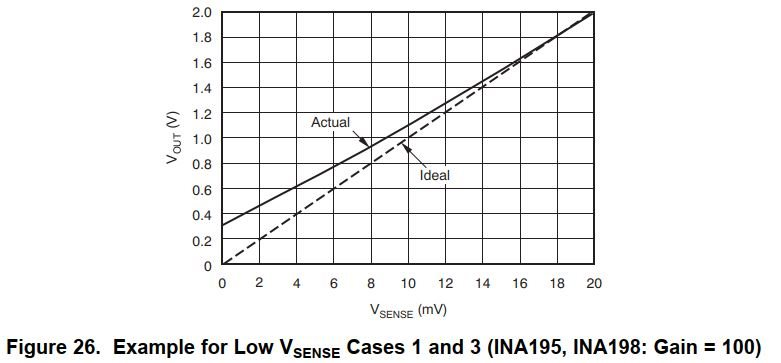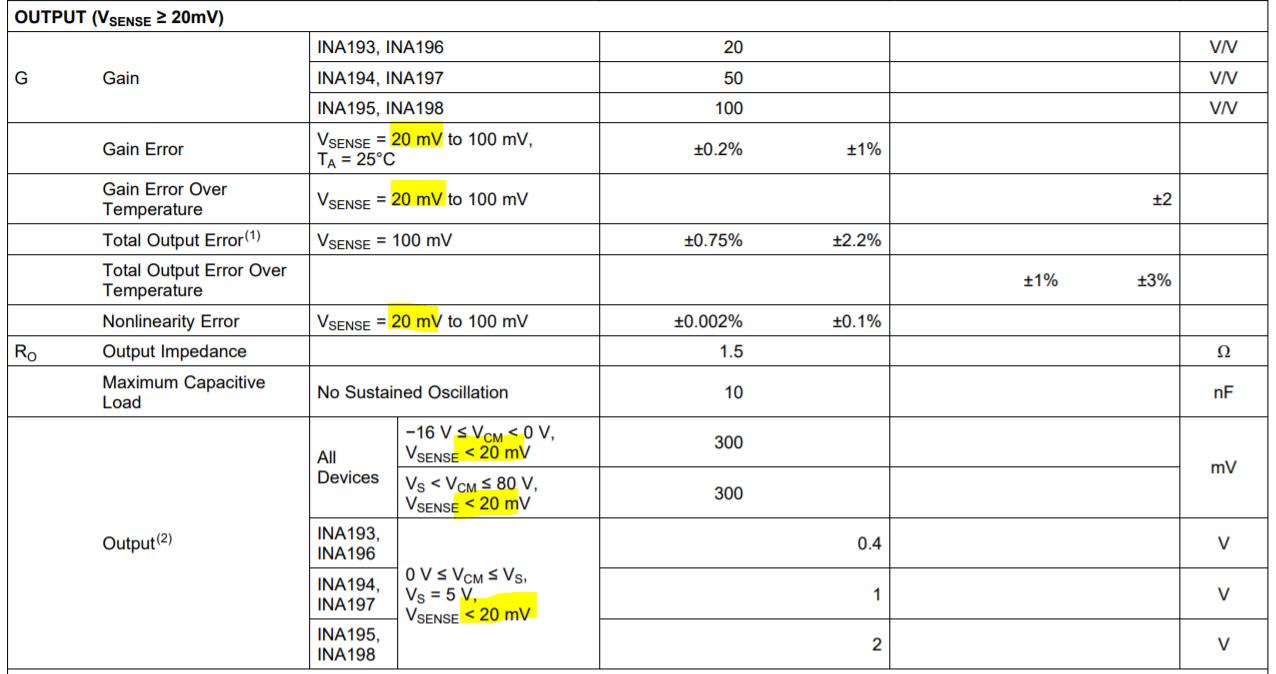If you have a related question, please click the "Ask a related question" button in the top right corner. The newly created question will be automatically linked to this question.

# INA198: INA198 non linearity

Part Number: INA198
Other Parts Discussed in Thread: INA180, INA293, INA290, INA240, INA190, INA186, INA196Hello Javier,

In Fig3, there is a problem with the linearity of the INA198 device output.

Q1> The measured current was tested in the Current Shunt Amplifer (INA198).

However, when the load is changed to 5V, the output voltage of the INA198 read by the ADC is not linearity.

Small current value due to fluctuation in 100 ~ 50 ohm load variable resistance value

And the current value of 50 ohm to 5 ohm is large.

Is there any way to correct non linearity?

Best Regards,
Jame, Shin• Hello Jame,

There is non-linearity because you are operating the device outside of the specified linear region (Vsense > 20mV). Your max Vsense is only around 18.18mV when variable resistor is 3-Ω. This creates a load of 5V/(3Ω+2.5Ω) = 0.909, which equates to 18.182 mV Vsense.

In order to resolve this, there are a couple options:

1. Use a larger shunt resistor.

2. Use a different current-sense amplifier which will have a larger linear output region such as the the INA240, INA190, INA186, INA290, INA293, or INA180.

Sincerely,

Peter

•Hello Peter,

Q1> Why are you saying Vsense> 20mV?

Vsense> 20mV , Is this related to non linearity?

By the way, are you referring to the X-axis values in Figure 26 in the data sheet?

(VR 3ohm + R 2.5ohm), Vsense is 19mV.

Q2> You said “which equates to 18.182 mV Vsense.” What is the result of the theory?

Perhaps, Do you say, V_Rshunt = I * R, 0.909 A * 0.2 ohm = 181.82 mV?

Q3> The Vsense range in the data sheet of IN 198 is shown below.

All specifications at TA = 25 ° C, VS = 12 V, VIN + = 12 V, and VSENSE = 100 mV, unless otherwise noted.

Also, Vsense: (typ) 0.15V (max) (Vs-0.2) / Gain, (12V-0.2) / 100 = 0.118 V analysis is not possible?

Q4> Fig3 of the previous post. What is the scope of Block Diagram Vsense?

TA = 25 ° C, VS = 5 V, VIN + = 5 V, and VSENSE =? (un know)

Wouldn't it be correct to change the Vsense value with this?

Q5> Peter said >  Use a larger shunt resistor.

Please explain in detail how large the shunt resistor is to solve non linearity ?

I didn't understand what you said.

i.e) For maximum current of 1.5A and maximum voltage into ADC of 3.3V(ADC VREFP),

with a multiplication factor of 100 for the INA198 the maximum voltage across the sense resistor is 3.3V/100 or 0.033V.

with a maximum current of 1.5A the maximum resistor is R = V/I  = 0.033/1.5 = 0.022 Ohm.  ( around  0.02 Ohm)

Q6> The PCB is already designed with INA198 and SOT23-5.

devices you suggested, only INA293, SOT23-5 are Pin to Pin.

Does using this device solve non linearity?

Best Regards,
Jame, Shin

• Hey Jame,

Q1. The device will behave linearly when Vsense > 20mV. This is what figure 26 and the specifications say. For Figure 26, the X-axis is Vsense in mV.

Q2. When the variable resistance is 3-Ω, the total resistive load is 3+2.5 = 5.5Ω. This creates a load current of 5V/5.5Ω = 909mA. Using Ohm’s Law, I calculate the shunt voltage to be Vshunt = I*R shunt = 0.909A*0.02Ω = 18.182 mV. Your schematic shows the shunt resistor to be 0.02Ω. Please correct me if the shunt resistor is actually otherwise.

Q3. The Vsense range is really dictated by the testing condition for gain error. This shows that the specified gain error and linearity will be achieved when Vsense > 20mV. This is why your current circuit is not accurate or linear: because you Vsense < 20mV.Q4. Given the initial schematic (“Figure 3”) in the initial post, the appropriate range of Vsense should be 20mV to (Vs-0.2)/Gain, which equates to {20mV to 31mV}.

Q5. If the shunt resistor is increased, then by Ohm’s law, so will the shunt voltage (Vsense). Thus if Vsense is bigger and then greater than 20mV, then the device will operate linearly. However the INA198 with Vs=3.3V will not accommodate your desired dynamic range. If you want to measure currents from 50mA to 1.5A, then this is a range of 1.5/0.05 = 30:1. The linear dynamic range of INA198 with Vs=3.3V is 31mV/20mV = 1.55:1. Since this range is smaller than 30:1, you will have to choose a different device that is not a part of the INA19x family.

This is because if you change to the INA196 (Gain = 20V/V), the largest possible linear dynamic range becomes [(3.3V – 0.2)/20]/20mV = 155mV/20mV = 7.75:1. This range is still smaller than your desired 30:1, so it will not work. So you will need another device.

Q5. The INA293 will easily solve your circuit issue of non-linearity because it has a larger linear output region.

Hope this helps.

Best,

Peter

• Hello Peter,

Q1> Does the INA293 have linearity and non-linearity graphs as shown in Figure26 of the INA198 device?

Q2> Are the INA298x3 device linearity and non-linearity ranges as shown below?

When VS : +3.3V ,

linearity : GND + 50mV ≤ VOUT ≤ VS – 200mV,   (Gain Error : (typ) ±0.02 %)

non linearity : GND ≤ VOUT ≤ 50mV

Best Regards,
Jame, Shin

• Hello Jame,

Q1. I'm not sure which graphs you're referring to, but the INA293 datasheet does not need them. The gain error and linearity are specified for range in the testing condition.

Q2. Gain error and linearity are specified under a set of global and specific testing conditions. The testing conditions for gain error at 0.15% max are VS = 5V, VCM = 48V, TA = 25°C, and (GND+50mV) <= Vout <= {Vs-200mV).

The gain error will change with temperature according to the gain error drift of ±10ppm/°C max.

Nonlinearity error should be for the same output region as the gain error, but only at 25°C.

Changing the VS or VCM will not significantly alter gain or nonlinearity errors.

I would recommend watching our training videos if you would like to learn more about our products.